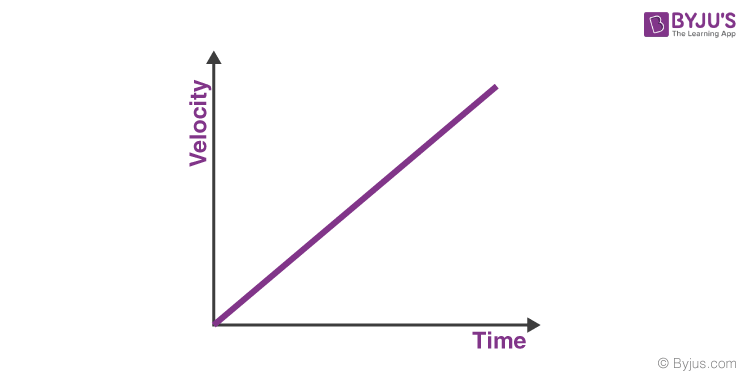# Draw a velocity-time graph for a uniform accelerated motion. What does its slope depict?

Draw a velocity-time graph for a uniform accelerated motion. What does its slope depict? In the velocity time graph as velocity changes at a constant rate with respect to time in uniformly accelerating motion, the graph would be a straight line with its slope indicating the amount of acceleration. This can be shown as: